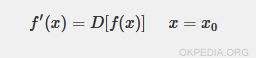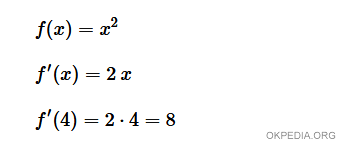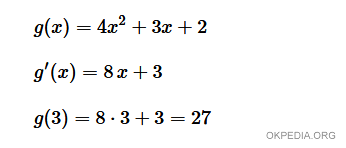# Find the derivative at a point in Python

To find the point derivative of a mathematical function f (x) using Python we use the derivative () function. It is a function of the misc module of scipy.

from scipy import misc
def f(x):
...
misc.derivative(f,x0)

• The first argument (f) is the function to be derived
• The second argument (x0) is the point.

The derivative() function calculates the first derivative of f(x) at the point x0.

What is the point derivative? It is the value of the derivative f '(x) at the point x = x0.## Examples

Example 1

Let's take the function f(x)=x2 as an example.

\$\$ f(x) = x^2 \$\$

To calculate the derivative of f (x) at the point x = 4.

>>> from scipy import misc
>>> def f(x):
return x*x
>>> misc.derivative(f,4)

The derivative() function calculates the value of the first derivative f '(x) at the point x = 4.

The output result is

8.0

The value of the first derivative of f(x) at the point x = 4 is equal to 8.

VerifyExample 2

In this example we define a more complex function.

\$\$ g(x) = 4x^2 + 3x + 2 \$\$

To calculate the derivative of g(x) at the point x = 3.

>>> from scipy import misc
>>> def g(x):
return 4*x**2+3*x+2
>>> misc.derivative(g,3)

The output result is

27.0

The value of the first derivative of g (x) at the point x = 3 is 27

Verifyhttps://how.okpedia.org/en/python/find-the-derivative-at-a-point-with-pythonReport us an error or send a suggestion to improve this page

Python Derivative